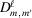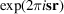International Tables for Crystallography (2006). Vol. F. ch. 13.2, pp. 269-274   | 1 | 2 | https://doi.org/10.1107/97809553602060000682

## Contents

• 13.2. Rotation functions  (pp. 269-274)
• 13.2.1. Overview  (p. 269) | html | pdf |
• 13.2.2. Rotations in three-dimensional Euclidean space  (pp. 269-270) | html | pdf |
• 13.2.2.1. The metric of the rotation group  (pp. 269-270) | html | pdf |
• 13.2.3. The rotation function  (pp. 270-272) | html | pdf |
• 13.2.3.1. Computing the rotation function  (p. 271) | html | pdf |
• 13.2.3.2. Plotting and sampling the rotation function  (p. 271) | html | pdf |
• 13.2.3.3. Strategies  (p. 272) | html | pdf |
• 13.2.3.4. Symmetry properties of the rotation function  (p. 272) | html | pdf |
• 13.2.4. The locked rotation function  (pp. 272-273) | html | pdf |
• 13.2.5. Other rotation functions  (p. 273) | html | pdf |
• 13.2.6. Concluding remarks  (p. 273) | html | pdf |
• Appendix 13.2.1. Formulae for the derivation and computation of the fast rotation function  (pp. 273-274) | html | pdf |
• A13.2.1.1. Euler parameterization  (p. 273) | html | pdf |
• A13.2.1.2. Thematrices  (pp. 273-274) | html | pdf |
• A13.2.1.3. Spherical harmonics  (p. 274) | html | pdf |
• A13.2.1.4. Spherical Bessel functions  (p. 274) | html | pdf |
• A13.2.1.5. Expansion of(p. 274) | html | pdf |
• A13.2.1.6. Expansion of the interference function  (p. 274) | html | pdf |
• References | html | pdf |
• Figures
• Fig. 13.2.2.1. Illustration of rotations defined by ( a ) the spherical polar angles (χ, ω, ϕ); ( b ) the Euler angles (α, β, γ)  (p. 269) | html | pdf |